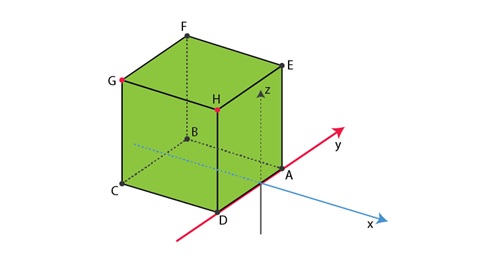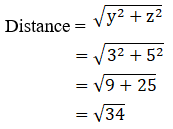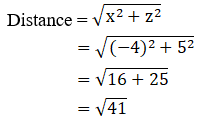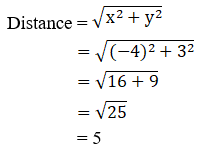• +91 9971497814
• info@interviewmaterial.com

# RD Chapter 28- Introduction to 3D coordinate geometry Ex-28.1 Interview Questions Answers

### Related Subjects

Question 1 :
Name the octants in which the following points lie:
(i) (5, 2, 3)
(ii) (-5, 4, 3)
(iii) (4, -3, 5)
(iv) (7, 4, -3)
(v) (-5, -4, 7)
(vi) (-5, -3, -2)
(vii) (2, -5, -7)
(viii) (-7, 2, -5)

Solution:

(i) (5,2, 3)

In this case, since x, y and z all three are positive thenoctant will be XOYZ

(ii) (-5,4, 3)

In this case, since x is negative and y and z are positive thenthe octant will be X′OYZ

(iii) (4,-3, 5)

In this case, since y is negative and x and z are positive thenthe octant will be XOY′Z

(iv) (7,4, -3)

In this case, since z is negative and x and y are positive thenthe octant will be XOYZ′

(v) (-5,-4, 7)

In this case, since x and y are negative and z is positive thenthe octant will be X′OY′Z

(vi) (-5,-3, -2)

In this case, since x, y and z all three are negative thenoctant will be X′OY′Z′

(vii) (2,-5, -7)

In this case, since z and y are negative and x is positive thenthe octant will be XOY′Z′

(viii) (-7,2, -5)

In this case, since x and z are negative and x is positive thenthe octant will be X′OYZ′

Question 2 :
Find the image of:
(i) (-2, 3, 4) in the yz-plane
(ii) (-5, 4, -3) in the xz-plane
(iii) (5, 2, -7) in the xy-plane
(iv) (-5, 0, 3) in the xz-plane
(v) (-4, 0, 0) in the xy-plane

(i) (-2, 3, 4)
Since we need to find its image in yz-plane, a sign of its x-coordinate will change
So, Image of point (-2, 3, 4) is (2, 3, 4)
(ii)(-5, 4, -3)
Since we need to find its image in xz-plane, sign of its y-coordinate will change
So, Image of point (-5, 4, -3) is (-5, -4, -3)
(iii) (5, 2, -7)
Since we need to find its image in xy-plane, a sign of its z-coordinate will change
So, Image of point (5, 2, -7) is (5, 2, 7)
(iv) (-5, 0, 3)
Since we need to find its image in xz-plane, sign of its y-coordinate will change
So, Image of point (-5, 0, 3) is (-5, 0, 3)
(v) (-4, 0, 0)
Since we need to find its image in xy-plane, sign of its z-coordinate will change
So, Image of point (-4, 0, 0) is (-4, 0, 0)

Question 3 : A cube of side 5 has one vertex at the point (1, 0, 1), and the three edges from this vertex are, respectively, parallel to the negative x and y-axes and positive z-axis. Find the coordinates of the other vertices of the cube.

Given: A cube has side 4 having one vertex at (1, 0, 1)
Side of cube = 5
We need to find the coordinates of the other vertices of the cube.
So let the Point A(1, 0, 1) and AB, AD and AE is parallel to –ve x-axis, -ve y-axis and +ve z-axis respectively.Since side of cube = 5
Point B is (-4, 0, 1)
Point D is (1, -5, 1)
Point E is (1, 0, 6)
Now, EH is parallel to –ve y-axis
Point H is (1, -5, 6)
HG is parallel to –ve x-axis
Point G is (-4, -5, 6)
Now, again GC and GF is parallel to –ve z-axis and +ve y-axis respectively
Point C is (-4, -5, 1)
Point F is (-4, 0, 6)

Question 4 : Planes are drawn parallel to the coordinates planes through the points (3, 0, -1) and (-2, 5, 4). Find the lengths of the edges of the parallelepiped so formed.

Given:

Points are (3, 0, -1) and (-2, 5, 4)

We need to find the lengths of the edges of the parallelepipedformed.

For point (3, 0, -1)

x1 = 3, y1 = 0 and z1 =-1

For point (-2, 5, 4)

x2 = -2, y2 = 5 and z2 =4

Plane parallel to coordinate planes of x1 and x2 isyz-plane

Plane parallel to coordinate planes of y1 and y2 isxz-plane

Plane parallel to coordinate planes of z1 and z2 isxy-plane

Distance between planes x1 = 3 and x2 =-2 is 3 – (-2) = 3 + 2 = 5

Distance between planes x1 = 0 and y2 =5 is 5 – 0 = 5

Distance between planes z1 = -1 and z2 =4 is 4 – (-1) = 4 + 1 = 5

Theedgesof parallelepiped is 5, 5, 5

Question 5 : Planes are drawn through the points (5, 0, 2) and (3, -2, 5) parallel to the coordinate planes. Find the lengths of the edges of the rectangular parallelepiped so formed.

Given:

Points are (5, 0, 2) and (3, -2, 5)

We need to find the lengths of the edges of the parallelepipedformed

For point (5, 0, 2)

x1 = 5, y1 = 0 and z1 =2

For point (3, -2, 5)

x2 = 3, y2 = -2 and z2 =5

Plane parallel to coordinate planes of x1 and x2 isyz-plane

Plane parallel to coordinate planes of y1 and y2 isxz-plane

Plane parallel to coordinate planes of z1 and z2 isxy-plane

Distance between planes x1 = 5 and x2 =3 is 5 – 3 = 2

Distance between planes x1 = 0 and y2 =-2 is 0 – (-2) = 0 + 2 = 2

Distance between planes z1 = 2 and z2 =5 is 5 – 2 = 3

The edgesof parallelepiped is 2, 2, 3

Question 6 : Find the distances of the point P (-4, 3, 5) from the coordinate axes.

Given:

The point P (-4, 3, 5)

The distance of the point from x-axis is given as:The distance of the point from y-axis is given as:The distance of the point from z-axis is given as:Question 7 : The coordinates of a point are (3, -2, 5). Write down the coordinates of seven points such that the absolute values of their coordinates are the same as those of the coordinates of the given point.

Given:
Point (3, -2, 5)
The Absolute value of any point(x, y, z) is given by,
√(x2 + y2 + z2)
We need to make sure that absolute value to be the same for all points.
So let the point A(3, -2, 5)
Remaining 7 points are:
Point B(3, 2, 5) (By changing the sign of y coordinate)
Point C(-3, -2, 5) (By changing the sign of x coordinate)
Point D(3, -2, -5) (By changing the sign of z coordinate)
Point E(-3, 2, 5) (By changing the sign of x and y coordinate)
Point F(3, 2, -5) (By changing the sign of y and z coordinate)
Point G(-3, -2, -5) (By changing the sign of x and z coordinate)
Point H(-3, 2, -5) (By changing the sign of x, y and z coordinate)

Todays Deals### RD Chapter 28- Introduction to 3D coordinate geometry Ex-28.1 Contributorskrishan

Name:
Email:

# Latest News# 9000 interview questions in different categories